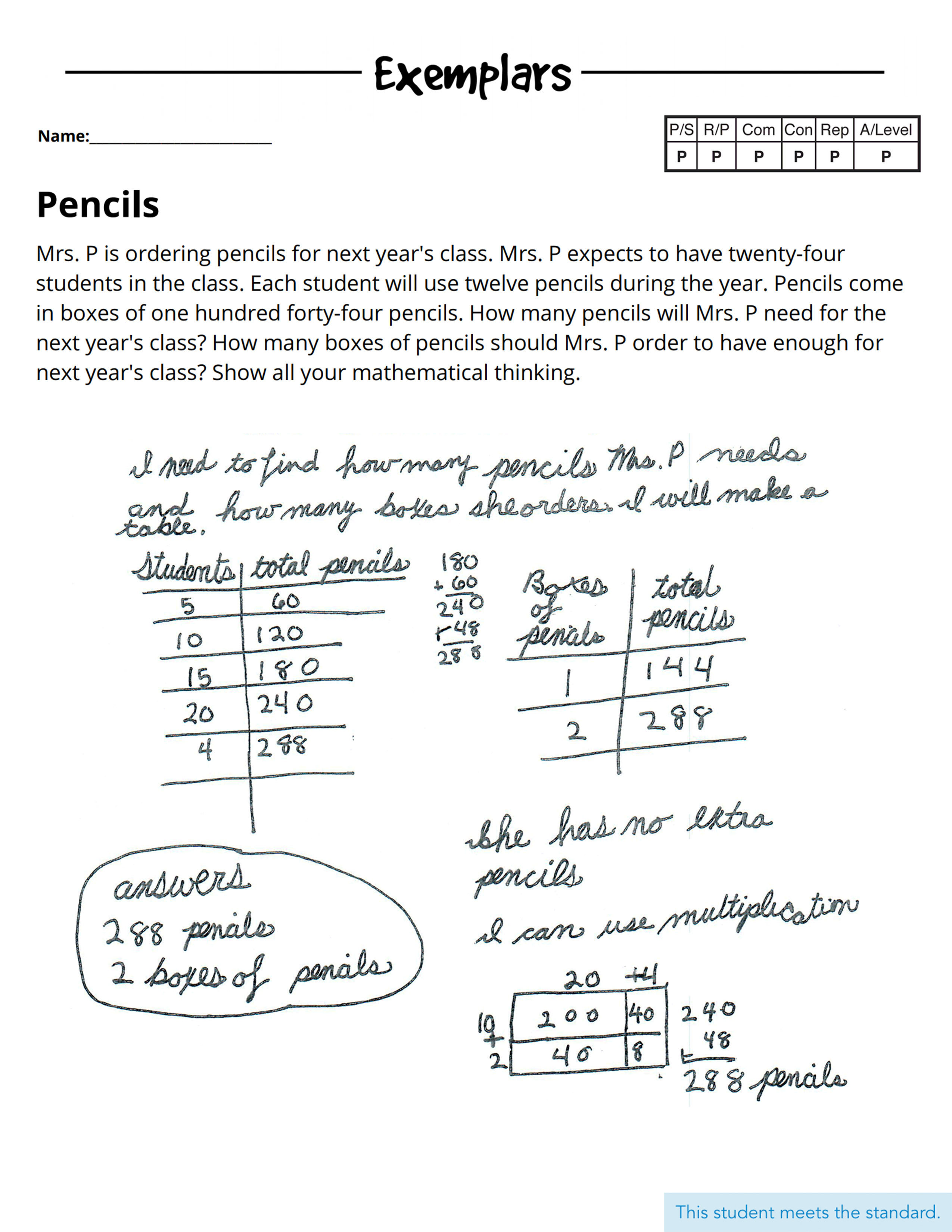# Assessing Student Skills in a Remote EnvironmentWith students working from home, are you finding it difficult to assess their mathematical understanding? That may be tied to the problems you’re asking them to solve. If you're only assessing procedural fluency, calculations, and the use of formulas while your students work remotely, you likely can’t see how they are arriving at their answers and if they truly understand the content—or if they’re getting help or searching the internet for solutions.

And that makes accurate formative and summative assessment a challenge: How can you be sure they’re getting the concept and not just getting an answer?

If you’re struggling to develop a clear picture of their progress, it’s time to switch up your approach so that it includes Exemplars performance tasks. Performance tasks require students to do more than just provide an answer in mathematics. They ask students to utilize an appropriate strategy, show their thinking, and explain their reasoning. No computer program can help a student develop the fundamental skills of problem solving: communicating with formal math language, generating representations, or making math connections. And no quick hint from a helper at home can reinforce what NCTM defines as Mathematical Process Standards (Problem Solving, Reasoning and Proof, Communication, Connections, Representations)—but performance tasks can.

When we ask students to demonstrate or “perform” their mathematical understanding by communicating their thinking and visualizing the relationship between quantities using representations, we are also asking them to think more deeply and to develop a richer understanding of the mathematics we want them to learn.

If we want fourth-grade students, for instance, to demonstrate understanding for the multiplication of whole numbers, we could simply ask them to complete several calculations. But how would we assess their conceptual understanding? How could we determine if their understanding of the concept is strong enough to be applied effectively in a real-world setting?

For these types of expectations, a performance task would ask students to construct a visual representation such as rectangular arrays, a number line, an area model, or even a table which clearly demonstrates conceptually the idea of multiplying two two-digit numbers. An additional expectation of a performance task would be for the student to use the math language associated with these concepts in their explanation of the process for completing the problem solving required in the task.

Just asking for an answer to a calculation leaves you without insights. By contrast, you need an assessment that fully reflects their level of understanding. Here’s an example of how you could do it through a performance task:

#### With Student Response & Assessment RationalesPROBLEM SOLVING: The student’s strategy of using tables to indicate the total number of pencils and boxes of pencils Mrs. P needs to order works to solve the task. The student’s answer, “288 pencils, 2 boxes of pencils,” is correct.

REASONING & PROOF: The student demonstrates an understanding of the underlying concepts of the task. The student is able to multiply two two-digit numbers, using strategies based on place value and the properties of operations.

COMMUNICATION: The student correctly uses the mathematical terms table, total, multiplication.

CONNECTIONS: The student makes the mathematically relevant observation, “She has no extra pencils.” The student uses the area model for multiplication to find 288 pencils.

REPRESENTATION: The student’s use of tables are appropriate to the task and accurate. The student provides correct labels and all entered data is correct. The student’s area model is appropriate for the task and accurate.

What might an Expert provide on a performance task that could demonstrate strong mastery of a concept? How about solving the task a second way? When a student recognizes the opportunity to solve the same problem utilizing different strategies and articulating connections between the different strategies, drawing conclusions about efficiencies of the different possibilities, the student is demonstrating Expert-level understanding.

Assessing students in a remote environment offers meaningful opportunities to evaluate more than procedural fluency—through the use of performance tasks like the ones that make up the Exemplars Library. Exemplars offers 800+ problem-solving performance tasks, assessment rubrics based on the NCTM Process Standards, and student work samples that demonstrate what it looks like to meet and not meet these expectations. When you use our tasks, your students practice performing in ways that simulate the use of real-world mathematics. You’re able to assess their math skills with accuracy and specificity. And all of it helps them grow as mathematical thinkers who strive not just for answers, but for rich, robust solutions.

Topics: